# Multiple choice questions on heat transfer for JEE Main and Advanced

Multiple choice questions with one or more answers

Question 1. A Cylinder of radius R made of a material having thermal conductivity K is surrounded by a parrallopied having inner hole of radius R and outer dimension of 3 R each. Thermal conductivity of parrallopied is 2K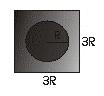The effective thermal conductivity of the system
a, K[2 + (π/9)]
b, K[2 + (π)]
c, K[2 - (π/9)]
d, 3 K

Question 2. Three black bodies are such that higher intensity wavelengths are in the ratio
λm1 : λm2 : λm3 = 1 :(21)1/2: (3)1/2
which of the these is true for the temperatures
a, T1 > T3 > T2
b, T1 > T2 > T3
c, T3 > T2 > T1
d, T3 > T1 > T2

Question 3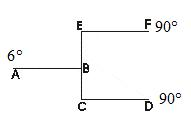All Rod are of equal length and equal area of cross-section. Find the temperature at point B
a, 45
b, 51.4
c, 50
d, 51

Question 4
Three identical rods are connected between two container contained at Temp. T1 and T2 (T1 > T2 ) .There are three possible way to join the rod both the container as show below.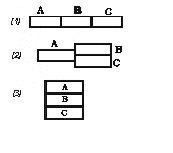Let Q1, Q2, Q3 are the rate of heat flow for these three figure respectively
when of these is true.
a, Q3 > Q2 > Q1
b, Thermal resistance is maximum in fig(a)
c, Q1 : Q3 = 1 : 9
d, All the above

A rod of thermal conductivity K and area A and length L is there. The initial temperature distribution along the rod is given by
T = T0 sin (π x / L)
where T0 is in ºC. Suppose both the end of the rod kept at 0ºC.

Question 5 which of these is the initial temperature distribution diagram

a.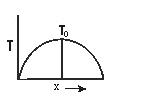b.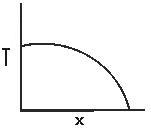c.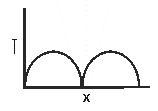d.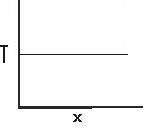Question 6 What is the initial temperature gradient at the end of rods
a, T0 π/L
b, T0π
c, T0 / L
d, T0/πL

Question 7 What is the initial heat current from the ends of the rods into the bodies making contact with its ends
a, πKAT0/L
b, KAT0/L
c, 3KAT0/L
d, 4KAT0/L

Question 8 What is the initial heat current at the center of rod
a, zero
b, KAT0/L
c, 2KAT0/L
d, 2KAT0/3L

Multiple choice questions with one or more answers
Question 9 Steady state temperature distribution along a rod is shown by which figure.
a.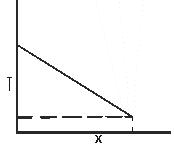b.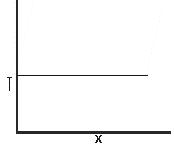c.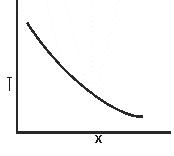d.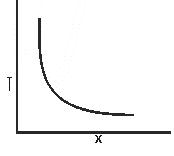Question 10 Which of these is true for a black body

a.(a = 1) , (e = 1)
c, frequency of radiation which is dominant is directly proportional to the temperature of the body
d, All of the above

Question 11
There are two bodies A & B they are having same surface and same mass. They are at temperature T at time t=0
Temperature of the surrounding is T0
Given (T > T0)
Let a1 & a2 are the absorptive power of the two surface and a1 > a2
which of these is true
a, Body A will cool faster than body B
b, Emissivity is greater for body B
c, Emissivity is greater for body A
d, Body B will cool faster than body A

Two sphere A & B are there of same material
sphere A is hollow sphere
sphere B is solid sphere
Radius is same for both the sphere
They are at same temperature T0

Question 12
Two statement are made about the above scenario
A) dT/dt at t = 0 will be same for the sphere
(B) Emissive power of both the sphere will be same in the beginning

(a) A & B both are correct
(b) Only B is correct
(c) Only A is correct
(d) None of them is correct

Question 13 if the temperature difference between sphere and surrounding is T for both the sphere which one is true
a. A will cool faster for all values of T
b. A & B both will cool at same rate for all values of T
c. A & B will have equal temperature at any instant
d none of the above

Question 14 if the sphere A radius is doubled then what will be ratio of emissive power of sphere A & B in the beginning
a E1:E2=4:1
b.E1:E2=1:4
c. E1:E2=2:1
d. E1:E2=1:2

Multiple choice questions with one or more answers
Question 15
A hot body whose temperature is T is kept in a room where temperature is T0.The hot body takes time t1 to cool from temperature T to T2,time t2 to cool from temperature T2 to T3,time t3 to cool from temperature T3 to T4

Also T > T2 > T3 > T4 > T0
and T - T2=T2 -T3=T3-T4=a
which one of the following is true
a. t1>t2>t3
a. t3>t2>t1
a. t1=t2=t3
d. None of the above

Question 16 if the temperature of the body is plotted against time which of the following curve will represent it
a.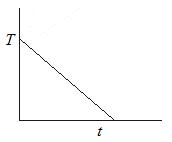b.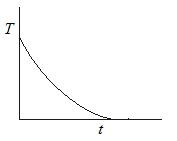c.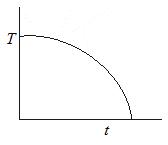d.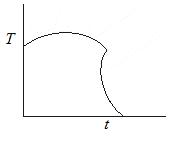Q-17 If the area is doubled and temperature is made halved what will be the ratio of power radiated
a E1:E2=4:1
b.E1:E2=1:1
c. E1:E2=1:4
d. E1:E2=1:2

Question 18 Suppose A body behaves like black body. When the temperature of blackbody increases, it is observed that the wavelength corresponding to maximum energy changes from λ to λ/2. Find the ratio of emissive power of the body at respective
temperature
a E1:E2=1:8
b.E1:E2=1:2
c. E1:E2=1:1
d. E1:E2=2:1

Question 19: Which of the following is the vm-T graph for a perfectly blackbody. Here vm is the frequency at maximum emission of radiation and T is the absolute temperature of the body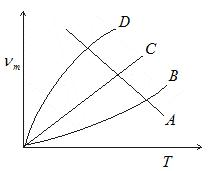Question 20
A composite slab is prepared by pasting three slab of thickness L1,L2,L3 and thermal conductivity K1,K2,K3.The slab have equal crossectional area. Find the equivalent thermal conductivity
a.K1K2K3(L1+L2+L3)/K2K3L1+K1K3L2+K1K2L3
b.K2K3L1+K1K3L2+K1K2L3/K1+K2+K3
c. K1+K2+K3/L1+L2+L3
d. L1+L2+L3/K1+K2+K3